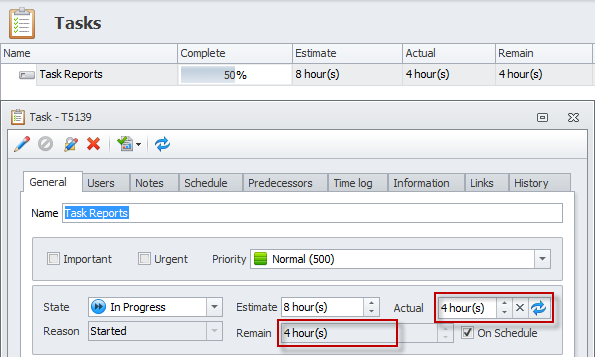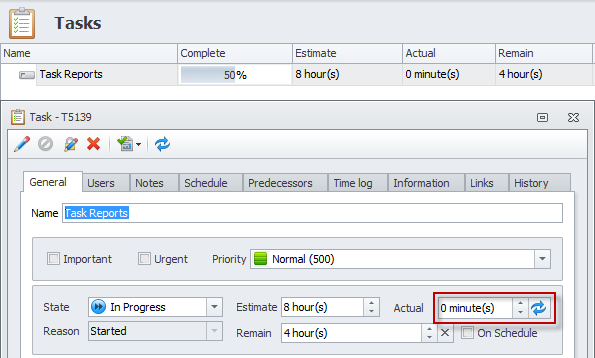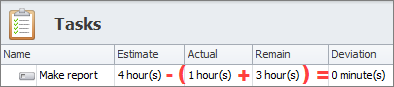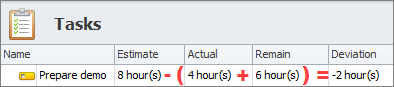Successful task completion relies on your ability to spot any possible troubles with task execution early through effective tracking tools. You should be always in control of task progress to complete your plan on-time and on-budget. In CentriQS time tracking system you can track task progress by using 'task completion formula' and be aware of ‘at risk’ tasks by using duration variance.

## Track Task Progress

'Complete' column shows task progress bar which lets users track task progress as percentage of task completion. The value of task completion is calculated by either of two formulas depending on the fields used for time tracking.

1. If both actual duration and remaining duration are used, task completion is calculated by formula:

Complete = Actual / (Actual + Remain) * 100

For example, estimated duration of task "Task Reports" is 8 hours, user spent 4 hours, and 4 hours remained, so the task is 50% complete: 4/(4+4)*100=50%2. If only remaining duration is used, task completion is calculated by formula:

Complete = (Estimate - Remain) / Estimate * 100

For example, estimated duration of task "Task Reports" is 8 hours, user thinks that 4 hours remained till task completion, so the task is 50% complete: (8-4)/8*100=50%## What is Duration Variance?

Duration variance answers the question: “Is the task taking more, exactly as much or less time than originally planned?” In other words it is the difference between estimated duration (which is baseline duration) and current estimated duration (which is the total of actual and remaining duration). Duration variance is used to know whether the task is taking more or less time than you originally planned. Our time tracking program helps analyze your project’s progress and employee performance up to now.

## Duration Variance Formula

Duration variance is calculated by the following formula: Estimate - (Actual + Remain) where ‘Estimate’ is estimated duration (baseline duration), ‘Actual’ is actual duration and ‘Remain’ is remaining duration. The result is displayed in the ‘Deviation’ field and may have zero, positive, or negative value.### Zero Deviation

Task duration variance is zero when 'Estimate' equals sum of 'Actual' and 'Remain'. Zero deviation usually means that everything goes as planned and no corrective actions needed. For example, if user feels that the task stays on the track, user can check the option “On Schedule”, so that our time tracking system can auto-calculate ‘Remain’ as the difference between ‘Estimate’ and ‘Actual’, i.e. if estimated duration of the task is 4 hours, user enters actual duration 1 hour that he or she has already spent, remaining duration will automatically become 3 hours, so deviation is 0.### Positive Deviation

Task duration variance becomes positive when 'Estimate' is greater than sum of 'Actual' and 'Remain'. For example, estimated duration of the task is 8 hours, but user who has spent 4 hours, decides that 2 hours are more than enough to close the task. So when the user enters “4 hours” into the ‘Actual’ field and “2 hours” into the ‘Remain’ field, ‘Deviation’ fields will automatically show “2 hours”.There are several reasons for positive deviation and corrective actions you can take depend on particular reason:

• If the task was initially overestimated and will actually take less time than it was planned, you can shift the schedule of future or successor tasks to earlier dates.
• If task executor skills were underestimated so he or she will close the task faster than it was expected, you can take it into account when estimating the duration of tasks assigned to this person.
• If the result of closed task is of lower quality that it was required by task owner or project sponsor, you can roll-back the task to state “In progress”.
• If task executor undervalues the task difficulty and makes wrong estimation of remaining duration, you can discuss the task with him or her and enter a more realistic value into the ‘Remain’ field.

### Negative Deviation

Task duration variance becomes negative when 'Estimate' is greater than sum of 'Actual' and 'Remain'. For example, estimated duration is 8 hours, but user who has spent 4 hours, decides that 4 hours are not enough to close the task and 2 additional hours will help greatly. So when the user enters “4 hours” into the ‘Actual’ field and “6 hours” (4 + 2 additional hours) into the ‘Remain’ field, ‘Deviation’ fields will automatically show “- 2 hours”.There are several reasons for negative deviation and corrective actions you can take depend on particular reason:

• If the task was initially underestimated and will actually take more time than it was planned, you can shift the due dates or schedule of next or dependent tasks to later dates.
• If task executor skills were overestimated so he or she is going to close the task later than it was expected, you can reassign the task to another user or assign more users to the task in order to help the task executor to complete it in time.
• If task executor overstates the task difficulty and makes wrong estimation of remaining duration, you can discuss the task with him or her and enter a more realistic value into the ‘Remain’ field.

## Track Tasks “At Risk”

The column “At Risk” shows which tasks should be paid more attention to. “At Risk” tasks are tasks which negative deviation is critical and requires corrective actions mentioned above. If negative deviation is not critical, then corrective actions may not be required. For example, if estimated duration of the task is 8 hours and deviation is 10 minutes only, in most cases it is not a problem.Column “Deviation %” shows how “Actual” + “Remain” deviates from “Estimate” percentagewise and lets our time tracking program define whether tasks with negative deviation are “at risk” or not. For example, estimated duration of the task is 8 hours, the total of actual and remaining duration is 10 hours, so deviation is -2 hours, which is -25%.By default, tasks with deviation which less than -10% become automatically become “at risk”. If 10% is too much or too little you can set your own Deviation from Estimate %. Icon of ‘at risk’ tasks turns yellow. If that is not enough to attract users’ attention, you can check highlight background option and select needed color.Additionally task owners are notified of “at risk” tasks by popup alerts and email. There is a subscription “At risk task event” that sends notifications once the ‘Deviation %’ property becomes less than -10%. If you don’t want to be notified of “at risk” tasks, you can deactivate this subscription.Next steps

{

Check out Task Management FEATURES

Watch Task Management Solution VIDEO OVERVIEW

Learn how to manage tasks in KNOWLEDGE BASE